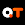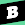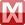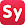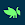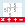# Gauthmath-Math Homework Solver

by GauthTech Pte. Ltd.
10,000,000+ installs

Android application Gauthmath-Math Homework Solver developed by is listed under category Education. According to Google Play Gauthmath-Math Homework Solver achieved more than 10,000,000 installs. Gauthmath-Math Homework Solver currently has 72,061 ratings with average rating value of 4.53. The current percentage of ratings achieved in last 30 days is 19.56%, percentage of ratings achieved in last 60 days is 39.12%. Gauthmath-Math Homework Solver has the current market position #11240 by number of ratings. A sample of the market history data for Gauthmath-Math Homework Solver can be found below. Last update on 2022-06-04.

Title: Gauthmath-Math Homework Solver GauthTech Pte. Ltd. Education Free Android
Total ratings: 72061 19.56% 39.12% 4.53
Installs (achieved): 10,000,000+ 22,590,363
5 star ratings: 61,232 1,851 1,383 916 6,639

### Country Rankings

Rank in category Education:

2021-10-17: Android application Gauthmath-Math Homework Solver achieved 10,000,000 installs. Android application Gauthmath-Math Homework Solver achieved 5,000,000 installs. Android application Gauthmath-Math Homework Solver achieved 1,000,000 installs. Android application Gauthmath-Math Homework Solver achieved 100,000 installs.

Total number of ratings
Total number of active users rated for Gauthmath-Math Homework Solver.

Total number of installs (*estimated)
Estimation of total number of installs on Google Play. Approximated from number of ratings and install bounds achieved on Google Play.

Average rating
Average rating value on Google Play. Given by active users of the application.